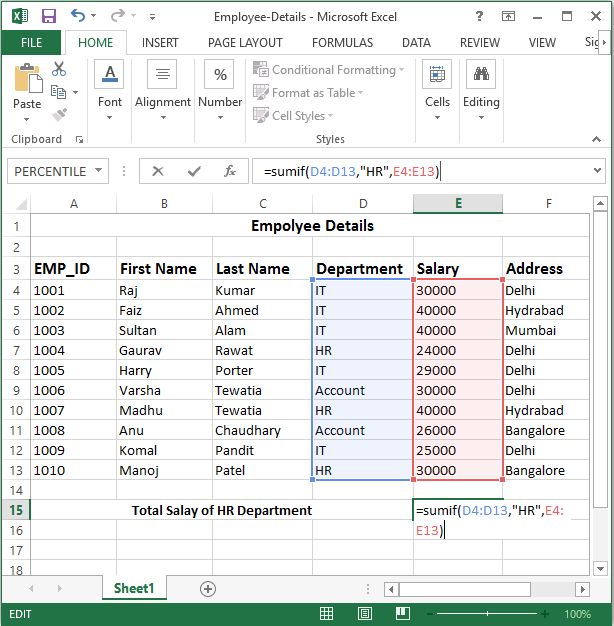# Sumif Function in Excel

## Sumif Function in Excel

The SUMIF function is a worksheet function that adds all numbers in a range of cells based on one criteria (for example, is equal to 2000). The SUMIF function is a built-in function in Excel that is categorized as a Math/Trig Function.

## Syntax

```=SUMIF(range,criteria, [sum_range])
```

SUMIF function has three main parts;

Range: It refers to range of cells that you want to evaluate to shortlist the cells that meet the given criteria.

Criteria: It refers to conditions that tell which cells are to be added. It can be a number or a text.

Sum _range: It provides the actual cells that are to be added. It is an optional argument. If we omit this part of the function the SUMIF function treats "range" as "sum_range" thus adds the cells of the range argument.

### See in Below example:

The company wants to know the total salary of the HR department. In this case, by applying the SUMIF function the company can add the salaries of all employees of HR department. You can see in below image.## Example

```=sumif(D4:D13,"HR",E4:E13)
```
• Range: D4:D13 is the range to be evaluated to shortlist the cells that belong to marketing department.
• Criteria: "HR" is the criterion to evaluate the range or to find out the cells that are to be added.
• Sum_range: E4:E13 is the sum_range that provides the actual values to be added based on the given criterion.